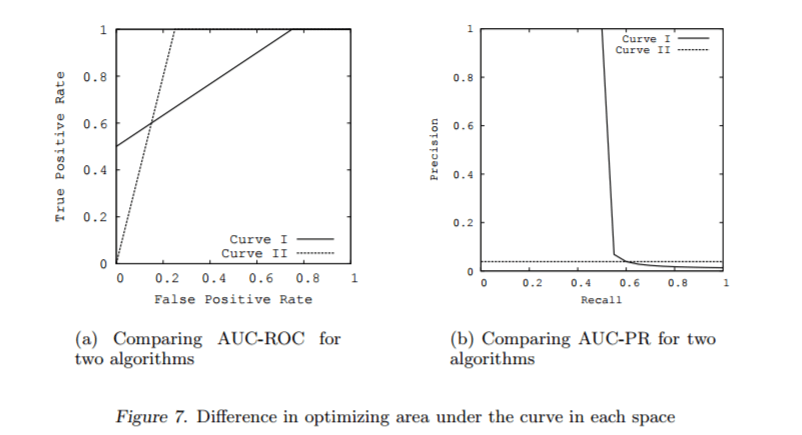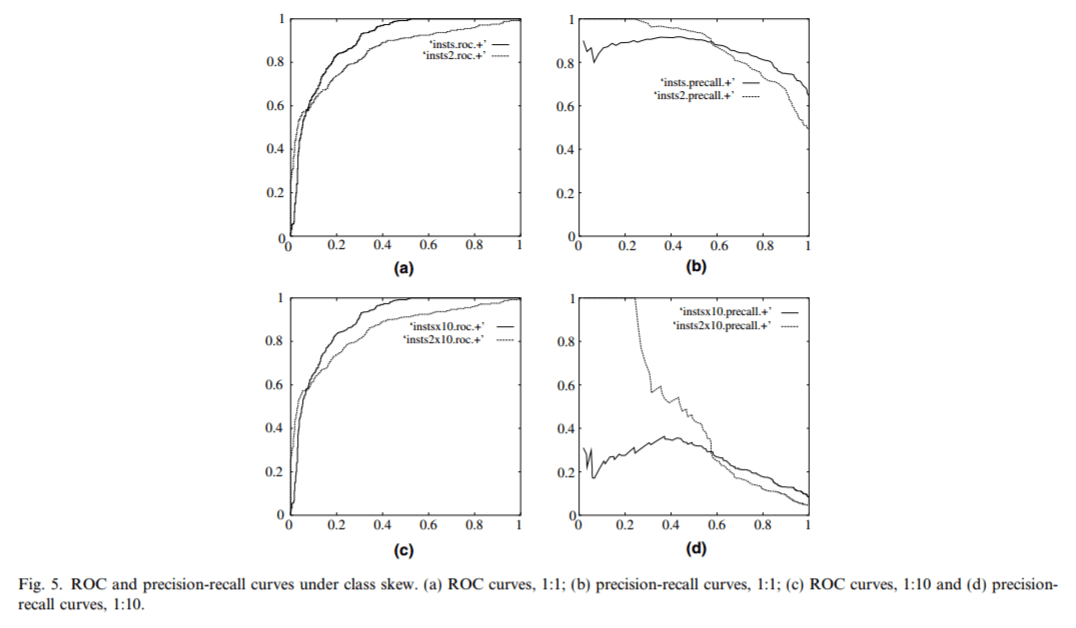# Handling Class Imbalance with R and Caret – Caveats when using the AUC

January 2, 2017
By

Want to share your content on R-bloggers? click here if you have a blog, or here if you don't.

In my last post, I went over how weighting and sampling methods can help to improve predictive performance in the case of imbalanced classes. I also included an applied example with a simulated dataset that used the area under the ROC curve (AUC) as the evaluation metric. In this post, I will go over some issues to keep in mind when using the AUC in the case of imbalanced classes and highlight another metric that is useful to examine: area under the precision-recall curve (AUPRC).

To quickly catch up, essential code from the previous post is below:

## Issues with using ROC for imbalanced classes

While using the AUC as an evaluation metric for classifiers on data with imbalanced classes is a popular choice, it can be a misleading one if you are not careful. Take the following example from Davis and Goadrich (2006). Below we see the model performance for two classifiers on an imbalanced dataset, with the ROC curve on the left and the precision-recall curve on the right. In the left example, the AUC for Curve 1 is reported in the paper as 0.813 and the AUC for Curve 2 is 0.875. So blindly choosing the best AUC value will choose Model 2 as the best. However, the precision-recall curve on the right tells a much different story. Here the area under Curve 1 is 0.513 and for Curve 2 it is 0.038. Due to Curve 1 having much better early retrieval compared to Curve 2, we see this massive discrepancy in the precision and recall performance between the two classifiers.Another example of how the ROC curve can be misleading comes from Fawcett (2005). Here, we have two datasets, one that has perfect balance between two classes (1:1) and the other has moderate imbalance (10:1). The column on the left shows the ROC curves of two classifiers for both datasets being identical, with the classifier represented by the dashed line having better early retrieval than the classifier represented by the solid line. Again, the precision-recall curve displayed on the right highlights a large discrepancy in performance between the two classifiers on the two datasets. In the case of balanced classes, both classifiers have consistently good precision and recall performance across all thresholds. On the imbalanced data, however, the classifier with better early retrieval has much better precision for lower values of recall.For these reasons, Saito and Rehmsmeier (2015) recommend examining the precision-recall curve as it is more explicitly informative than a ROC curve in the case of imbalanced classes. We can calculate the area under the precision-recall curve for our 5 classifiers using the PRROC package in R to create a custom function, `calc_auprc`. Here, we see a similar story to what was found with the AUC metric in the last blog post; we have better performance for the weighted model, followed by the sampled models, with the original model coming in last. However, now the difference in performance is much more apparent.

We can dig deeper into these results by actually plotting the precision-recall curves. Below, we see that both up sampling and weighting offer the best precision and recall performance depending on the threshold that is chosen, while the original classifier is essentially the worst performing across all thresholds. For example, the weighted classifier simultaneously has a recall of 75% and a precision of 50%, resulting in an F1 score of 0.6, while the original classifier has a recall of 75% and a precision of 25%, resulting in an F1 score of 0.38. In other words, when both classifiers create their predictions and use a particular threshold to obtain hard classifications, they both correctly identify 75% of the cases that are actually in the minority class. However, the weighted classifier is more efficient in these predictions, in that 50% of the observations predicted to be in the minority class actually are, while for the original classifier, only 25% of the observations predicted to be in the minority class actually are.## Implementing the area under the precision-recall curve metric in caret

One might imagine wanting to choose hyperparameters of a classifier by using the area under the precision-recall curve rather than the AUC, as some combinations of hyperparameters for a given model might have better early retrieval performance compared to others. It is incredibly easy to create a custom summary function in caret to allow you to do this by combining the code for the `calc_auprc` function with these instructions found in the caret documentation.

This custom summary function is implemented below, along with code to re-run the original model now using area under the precision-recall curve as the evaluation metric. Here, we see that the original model implementation has the exact same results on the test set for the area under the PR curve, regardless of whether we build the model using the area under the ROC curve or the area under the precision-recall curve. This is because both select the same combination of hyperparameters to build the final model on. Note that this may not always be the case, especially if you decide to use the 1-SE rule when choosing the hyperparameters in order to encourage a more parsimonious solution.

## Final thoughts

The area under the precision-recall curve can be a useful metric to help differentiate between two competing models in the case of imbalanced classes. For the AUC, weights and sampling techniques may only provide modest improvements. However, this improvement typically impacts early retrieval performance, resulting in a much larger gain in the overall precision of a model. In conjunction with trying weighting or sampling, it is also recommended to avoid relying solely on the AUC when evaluating the performance of a classifier that has imbalanced classes as it can be a misleading metric. The code above shows how easy it is to use the precision-recall curve, a more sensitive measure of classification performance when there are imbalanced classes.

edit (1/3/17): Graciously pointed out to me by Max Kuhn, a recent release of caret allows you to use the `prSummary` function rather than a custom function in `trainControl` to calculate the area under the precision-recall curve. Additionally, the `confusionMatrix` function has a mode argument that will focus on precision and recall (rather than sensitivity and specificity). See this page for more information.

R-bloggers.com offers daily e-mail updates about R news and tutorials about learning R and many other topics. Click here if you're looking to post or find an R/data-science job.
Want to share your content on R-bloggers? click here if you have a blog, or here if you don't.